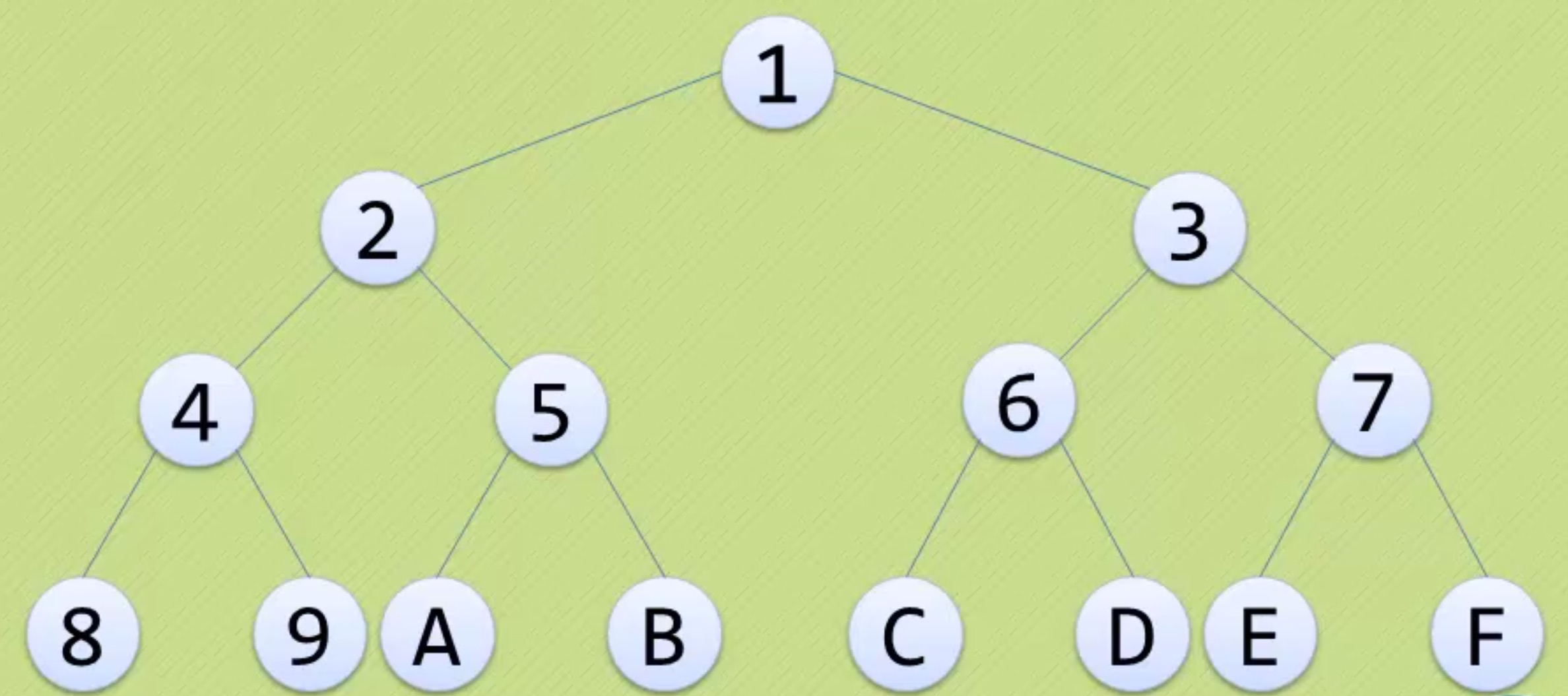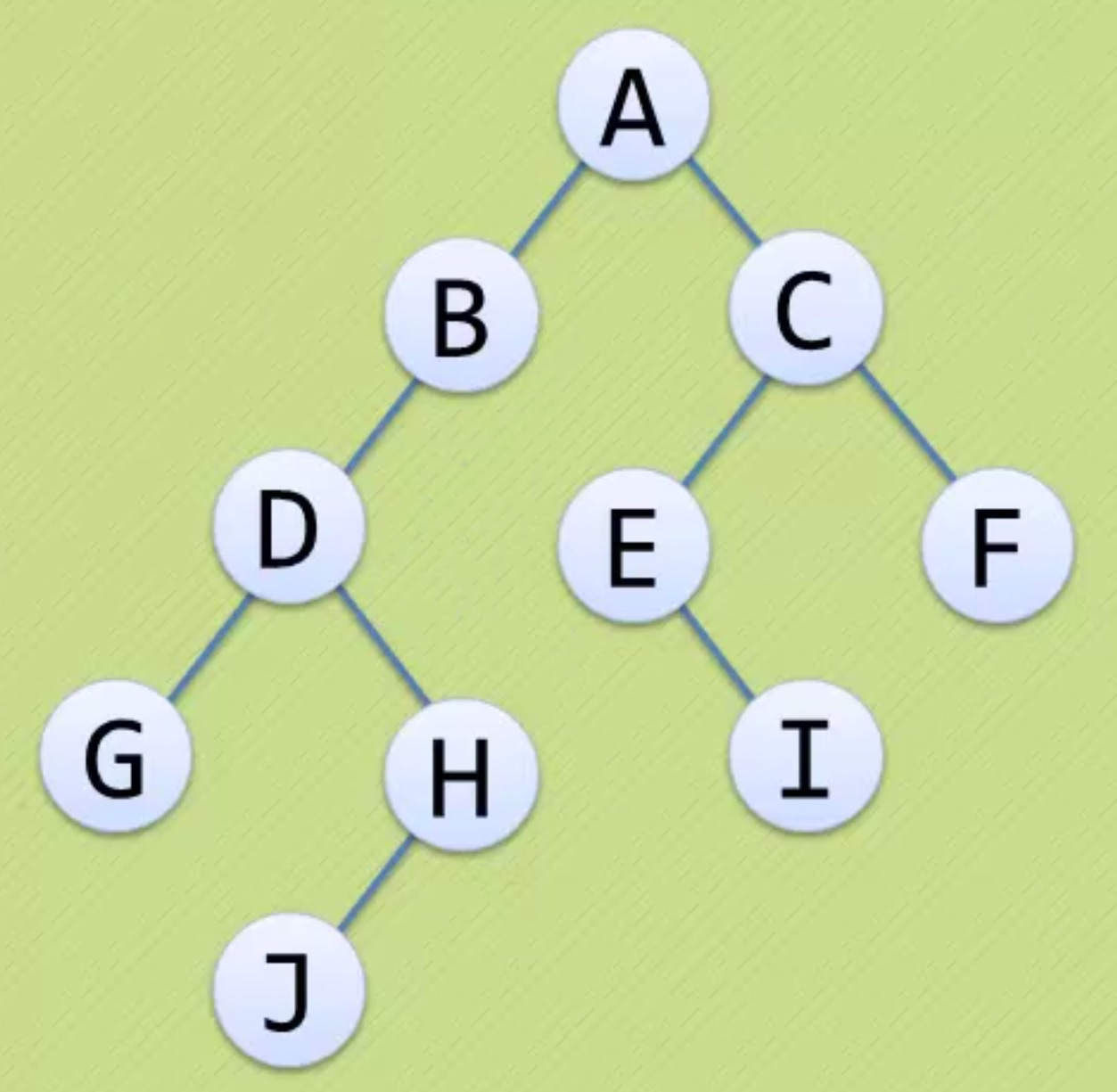# 每一层最大的节点数• 在二叉树的第 i 层，至多有$2^{i-1} (i \geq 1)$ 个结点
• 上面这张图中
• 第一层$2^0=1$个结点
• 第二层$2^1=2$个结点
• 第三层$2^2=4$个结点
• 第四层$2^3=8$个结点

# 从深度判断节点数• 深度为 k 的二叉树，至多有$2^{k}-1 (k \geq 1)$ 个结点。
• 也就是 K 层二叉树，满了也就是$2^{k}-1 (k \geq 1)$ 个结点。
• 一层$2^1-1=1$个结点
• 二层$2^2-1=3$个结点
• 三层$2^3-1=7$个结点
• 四层$2^4-1=15$个结点

# 树中度的关系• 度是每个结点包含的孩子数
• 度为2表示这个结点既有左子树，也有右子树
• 度为1表示这个结点可能只有左子树、或者只有右子树
• 度为0表示这个结点没有孩子，也称之为终端结点

• 度为2：A、C 和 D，共3个，记作 n2
• 度为1：B、H 、E，共3个，记作 n1
• 度为0：G、J、I、F，共4个，记作 n0
• 结点的总数就是n2+n1+n0=3+3+4=10个结点

• 结点和结点之间的连接数，也就是那条黑线，总是为总节点数-1
• 并且这个总节点数-1总是等于 $n1+2\times n^{2}$
• 所以$n-1=n1+2\times n2$
• 所以$n0+n1+n2-1=n1+n2+n2$
• 所以$n0=n2+1$
• 所以，度为0的结点数等于度为2的结点数+1

# 尾巴

-------------The End-------------